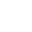# Shortest path problem with Excel solver

In today’s post, you will learn how you can use Excel for solving the shortest path problem. The shortest path problem is a fundamental optimization problem with a massive range of applications. It is used for example in logistical problem solving, project management, and routing – to only mention a few. In addition, it is a brilliant puzzle to improve your computational thinking! Just as the name states, the objective in the shortest path problem is to get from start to goal by minimizing the distance. Here is a simple network, where we can easily work out that the minimal distance from A to F is achieved by using node C -> E -> D, over a distance of 20.In reality, the distance between nodes is rarely pure physical distance nor a single number, but a combination of different variables that contribute to a number which represents the resources used to get from one node to another. For example, when a navigator plans your route, the route is calculated using a very similar principle. It takes into count things such as the physical distance, traffic, condition of the roads, and speed limits. These are then combined to represent an approximated time for moving between locations (nodes).

In a small dataset it is relatively easy to work out the shortest path without an algorithm simply by calculating all different possibilities by hand, but as the network grows this will become nearly impossible to do manually. Therefore, we need a generic solution that we can apply for a network of any scale.

Let’s create a new sheet where we will work out the shortest path for this particular network. We will start by mapping all the different routes with their respective distances.

From node A we can move to B and C, over a distance of 12 and 10.

And following the same rules, we can map the full network:

Note that backtracking is allowed, so we need to map certain routes in reverse as well. For example, the start of the path might be A -> B -> D or A -> C -> D -> B.

Here we have ignored some obvious ones for backtracking to scale it down, but generally it is not a good practice unless the route is explicitly a one-way path.

Create an empty column next to “Distance”. These cells will serve as binary variables when we solve the shortest path, marking the correct route.

Since we know that column “Go” will mark the correct routes with the number one, we create another column that will output the route in a more readable format.

If the correct route starts from A -> B, the formula will simply output “A to B”, otherwise it will stay blank. Copy the formula to all cells below.

Now we need to create a new table for the constraints that we will pass into the solver. In the first column, we will map all the nodes. In the “Constraints” column we will set up a function that will tell the solver our constraints. Since we are looking for a single route. Type = SUMIF(\$A\$2:\$A\$26;H2;\$D\$2:\$D\$26) – SUMIF(\$B\$2:\$B\$26;H2;\$D\$2:\$D\$26)

I know, it may look complicated, but all the functions does is return 1 if there is a used route going out of the node, 0 if there is a used route coming in and out of the node, and -1 if there is a route coming in but not out. It will return 0 as well if there is no route coming in nor out. Copy the formula to all cells below.

Since we need to tell the solver what our constraints should be equal to, we will add one more column where we type these values.

Before solving, we sure want to know the total distance as well, and not only the correct route, so we set up an objective function with SUMPRODUCT that sums the distances for the used routes, (SUMIF works fine as well)

Type =SUMPRODUCT(D2:D26;C2:C26)

Now we are all set up for solving the shortest path! Open up the solver – plugin. If you don’t have it installed go to File -> More -> Options and choose Add-ins. Click “Go” and select “Solver Add-in” from the menu. The solver Add-in will then appear in the toolbar, under the Data-tab.

In solver, we now need to assign the objective function and the changing variables. We will choose “Min” for minimizing and Simplex LP method for solving.

At last, click “Add” to assign the constraints. Set the Constraints in column I equal to the values in column J.

Click Ok and then Solve to get the answer!

We can now see the route for the shortest path in column E. The total distance for the path is 27.

If you have any questions, drop a comment below! 🙂

Learn the SUMIF-function (from Excel Essentials)

Learn the SUMPRODUCT-funtion (from Excel functions guide)

#### 2 thoughts on “Shortest path problem with Excel solver”

1.Daniel says:

Mind blowing. Just when you thought you knew excel!

2.First Last says:

Glad that you found a new perspective to Excel 🙂 In fact, many linear programming principles can be applied in Excel with some “fancy” tricks like using the solver!

/Emil

### More blog postsTired of Googling?